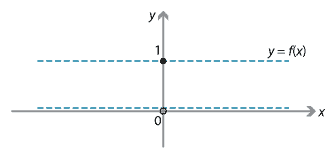## Content

### Function notation

A function is a rule for transforming an object into another object. The object you start with is called the input, and comes from some set called the domain. What you end up with is called the output, and it comes from some set called the codomain. There is a standard and very convenient notation for functions.

For example, we write the function $$y=x^2$$ as $f(x)=x^2.$ This is read as '$$f$$ of $$x$$ is equal to $$x^2$$'. To calculate the value of the function for some input $$a$$, we simply substitute $$a$$ for $$x$$ in the formula for $$f$$. For example, for this function we have $f(3) = 3^2=9, \quad f(0)=0, \quad f(-2)=4, \quad f(a)=a^2, \quad f(x+2)=x^2+4x+4.$ We are now distinguishing between the function $$f(x)$$ and its graph $$y=f(x)$$. A function can be given by a rule such as $f(x)=\dfrac{x^3\sin x}{(x-2)^2}, \quad\text{for } x\neq 2.$

We can easily calculate values of this function, even though drawing its graph may be quite difficult. This new way of writing functions is called function notation, and was introduced to mathematics by Leonhard Euler in 1735. It is now completely standard.

##### Exercise 2
For the function $$f(x)=\dfrac{x^2}{2} + x$$, find
1. $$f(3)$$
2. $$f(a + h)$$.

In general, a function should be specified by giving both the rule and the domain. It is not essential to draw the graph of the function, but very often the sensible first step in solving an algebraic problem or a problem using calculus is to draw the graph of the function.

#### Non-standard functions

Most functions encountered in secondary school mathematics are easily graphed.

In the late 19th century, when these ideas were being seriously considered for the first time, examples such as the following were introduced: $f(x) = \begin{cases} 1 &\text{if } x\in\mathbb{Q}, \\ 0 &\text{if } x\in\mathbb{R}\setminus \mathbb{Q}. \end{cases}$ Yes, this is a function! For example, we have $f(0)=f(3)=1 \quad\text{and}\quad f(\sqrt{2})=f(\pi)=0.$

It is not possible to draw the graph of the function, except perhaps as follows.At first, this type of example was dismissed by many mathematicians. But such examples turned out to be very important in finding the correct definitions of continuity and differentiability in advanced calculus (known as analysis).

Next page - Content - Finding domains and ranges